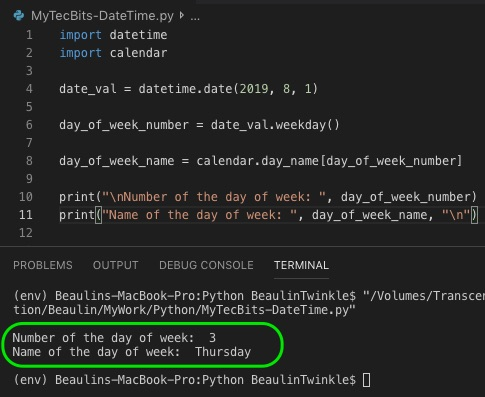# How to get day of week for a specific date in Python?

In Python programming, sometimes, we need to get the day of week of a specific date in name or number. Python has an inbuilt method for this. You can use the weekday() function of datetime module. This function returns the day-of-week as an integer from 0 to 6 representing Monday to Sunday.

## To get the number of the day of week

As mentioned earlier, use the weekday() method of datetime module to get the weeks day in integer value. Here is an example.

```import datetime

date_val = datetime.date(2019, 8, 1)

day_of_week = date_val.weekday()

print("\nDay of week: ", day_of_week, "\n")
```

## To get the name of the day

In the previous example we have seen how to get week day in integer value. Now we will see how to get the day name. For this we need to use the calendar module’s date_name attribute. date_name is an array of days of the week. Here is an example of using it.

```import datetime
import calendar

date_val = datetime.date(2019, 8, 1)

day_of_week_number = date_val.weekday()

day_of_week_name = calendar.day_name[day_of_week_number]

print("\nNumber of the day of week: ", day_of_week_number)
print("Name of the day of week: ", day_of_week_name, "\n")
```

## Reference

### Related Articles & Tools

This site uses Akismet to reduce spam. Learn how your comment data is processed.